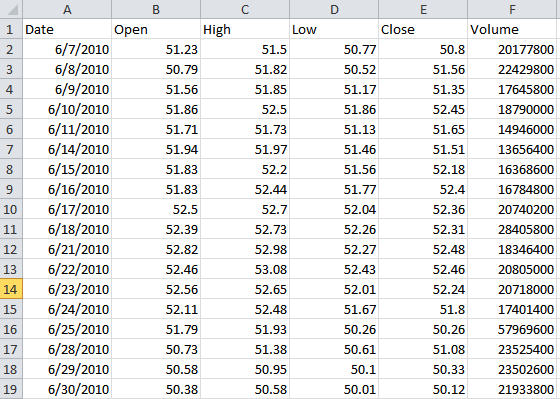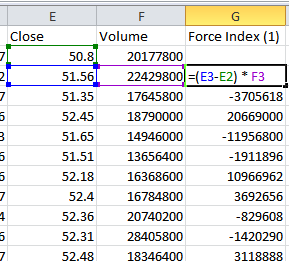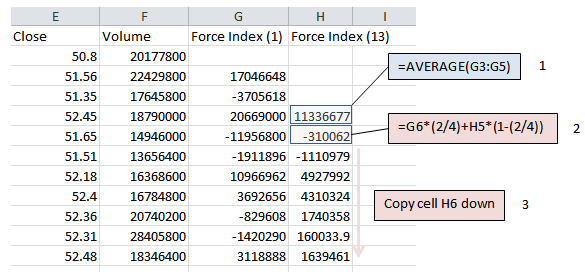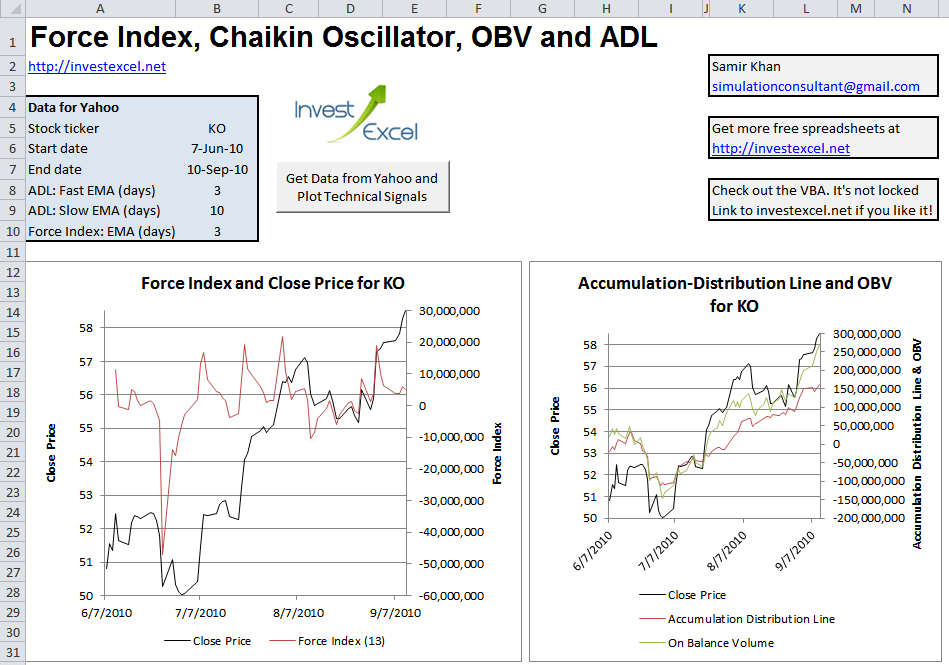# Force Index for Technical Traders

The Force index gauges whether bulls or bears are shaping the market, and helps you identify trends and short-term pullbacks.

Developed by Alexander Elder, the Force index marries together price, direction and trading volume to gauge market sentiment. When used with other indicators, the Force index is a powerful tool for confirming trends, short-term pullbacks and more.

The 1-period Force index is given by this formula:

Force index (1) = (Current Close – Previous Close) x Current Trading Volume

The price difference governs the direction of the market and has a smaller effect on market momentum. However, the trading volume is a major influence on market momentum.

Force index (1) can be rather choppy, so it’s usually smoothed with an exponential moving average of varying length. For example, a 13-period Force Index is simply a 13-period exponential moving average of the 1-period Force Index.

However, other scales of action can be revealed with a longer or shorter time window for the moving average. Some traders, for example, employ a 60-day moving average of the 1-dayForce Index.

## How to Interpret the Force Index

If the Force Index is

• negative, the market is bearish – the more negative the value, the greater the pessimism of bears.
• positive, the market is bullish – the more positive the value, the greater the optimism of bulls.
• near zero, the market is neutral – traders may want to sit on the sidelines.

## Divergences

If the Force Index is trending upwards, while the price continuously falls, then prices may reverse travel and start rising.

However, if the Force index falls day-by-day while prices rise, then a reversal may be forthcoming – the market could go into a downtrend.

## Identify Short-Term Pullbacks

Traders often use the Force Index to identify short-term pullbacks. For example, let’s say that a 25-day Relative Strength Index predicts a long-term rising market. IF a 2-day Force index is negative, the market may be at a temporary bottom.

In this case, you may want to set a buy order that’s higher than the maximum price on the day that the two-day EMA drops below zero

## Calculate the Force Index in Excel

Here’s a step- by-step guide to calculating this remarkable oscillator.

Step 1: Acquire your historical market data, and put it in a spreadsheet like so. Note that the close price is in Column E and the volume is in Column F.Step 2: Calculate the 1-day Force index in Column G. This would simply be (E3-E2) x F3Step 3: Assuming that you want to calculate a 3-day Force index, you’ll need to calculate the 3-day EMA of the data in Column G.

• In cell H5, first calculate the average of the first three values of Force Index (1). This is simply =AVERAGE(G3:G5)
• Then in cell H6, enter =G6*(2/4)+H5*(1-(2/4))
• Finally, copy cell H6 down## Automatically Chart Four Technical Indicators

This macro-powered Excel spreadsheet plots the Force index, Chaikin Oscillator, Accumulation-Distribution Line and On-Balance Volume for your chosen company. It downloads historical market OHLCV data from Yahoo Finance, and programmatically generates all the calculations and charts.Simply enter a ticker symbol, two dates, the size of the EMA windows and click a button – all the plots and calculations update automatically.

Some clever behind-the-scenes coding does all the hard work. The VBA is fully viewable and editable.

If you like this free tool and want to motivate me to develop more free spreadsheets, please consider linking to https://investexcel.net.